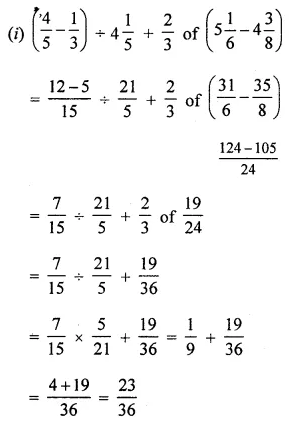# ML Aggarwal Class 7 Solutions for ICSE Maths Chapter 2 Fractions and Decimals Check Your Progress

## ML Aggarwal Class 7 Solutions for ICSE Maths Chapter 2 Fractions and Decimals Check Your Progress

Question 1.
What fraction is 270 gram of 3 kilograms?
Solution: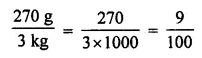Question 2.
Simplify the following:Solution:Question 3.
A shirt was marked at ₹ 540. It was sold at $$\frac { 3 }{ 4 }$$ of the marked price. What was the sale price?
Solution: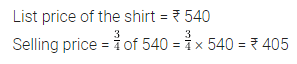Question 4.
In a class of 56 students, $$\frac { 1 }{ 4 }$$ are in blue house and $$\frac { 3 }{ 14 }$$ are in yellow house. Out of the remaining, $$\frac { 1 }{ 3 }$$ are in the greenhouse and the rest are in the red house. Find the number of students in each house.
Solution:Question 5.
Rohit bought a motorcycle for ₹ 36000. He paid $$\frac { 1 }{ 6 }$$ of the price in cash and the rest in 12 equal monthly installments. Find the amount he had to pay every month.
Solution: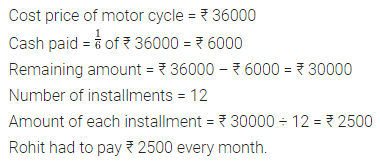Question 6.
Mr Mukerjee gave $$\frac { 5 }{ 14 }$$ of his money to his son, $$\frac { 2 }{ 3 }$$ of the remainder to his daughter and the rest to his wife. If his wife got ₹ 36000, what was that total amount?
Solution:Question 7.Solution: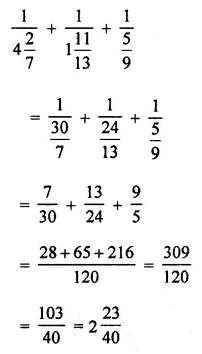Question 8.
Convert the following numbers to fractions (in simplest form):
(i) 0.025
(ii) 0.876
(iii) 4.3125
Solution: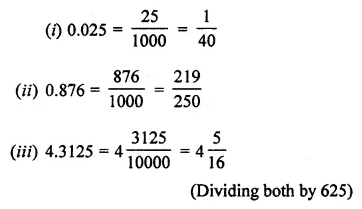Question 9.
Write the following fractions as decimals:
(i) 1$$\frac { 3 }{ 8 }$$
(ii) $$\frac { 47 }{ 125 }$$
(iii) 2$$\frac { 9 }{ 40 }$$
Solution:Question 10.
By how much does the sum of 17.443 and 29.657 exceed the sum of 13.687 and 18.548?
Solution: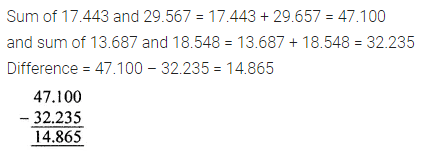Question 11.
Simplify the following:
(i) 4.27 × 0.036
(ii) 0.09 × 1.04
(iii) 1.32 ÷ 0.8
(iv) 0.7038 ÷ 0.34
Solution: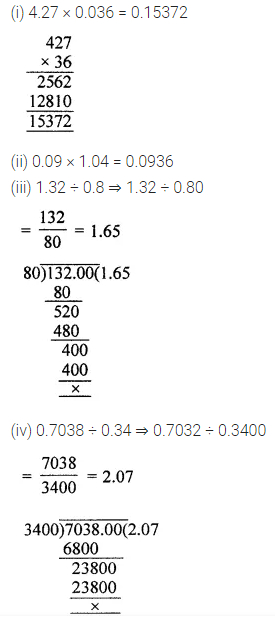Question 12.
If one kg of rice costs ₹ 52.70, then find the cost of 12.5 kg rice.
Solution:Question 13.
A piece of cloth is 24.5 m long. How many pieces, each of length 1.75 m, can be cut from it?
Solution:Question 14.
The product of two decimal numbers is 1.599 and one of them ¡s 0.65, find the other.
Solution:Question 15.
Simplify the following: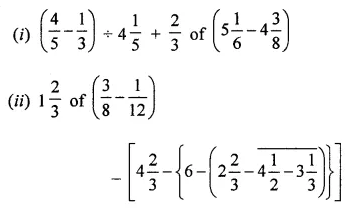Solution: